Скачать презентацию agi15 potapov tutorial slides

agi15_potapov_tutorial_slides.ppt

• Размер: 6.4 Mегабайта
• Количество слайдов: 61

## Описание презентации Презентация agi15 potapov tutorial slides по слайдам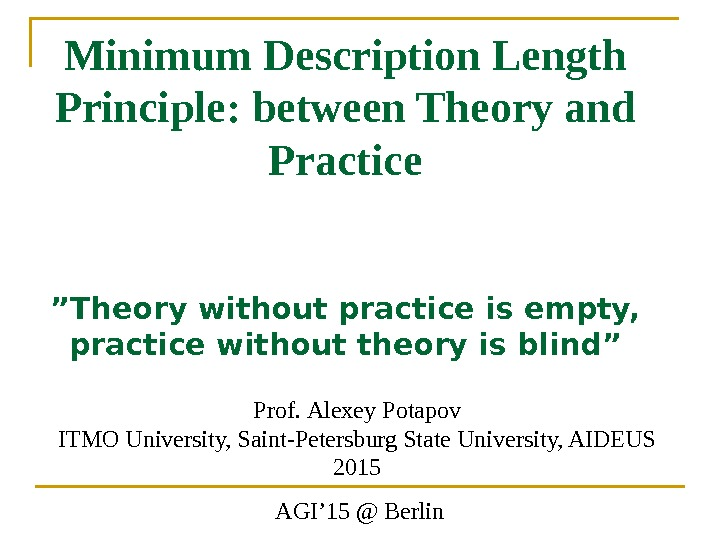Minimum Description Length Principle: between Theory and Practice ”Theory without practice is empty, practice without theory is blind” Prof. Alexey Potapov ITMO University, Saint-Petersburg State University, AIDEUS 2015 AGI’ 15 @ Berlin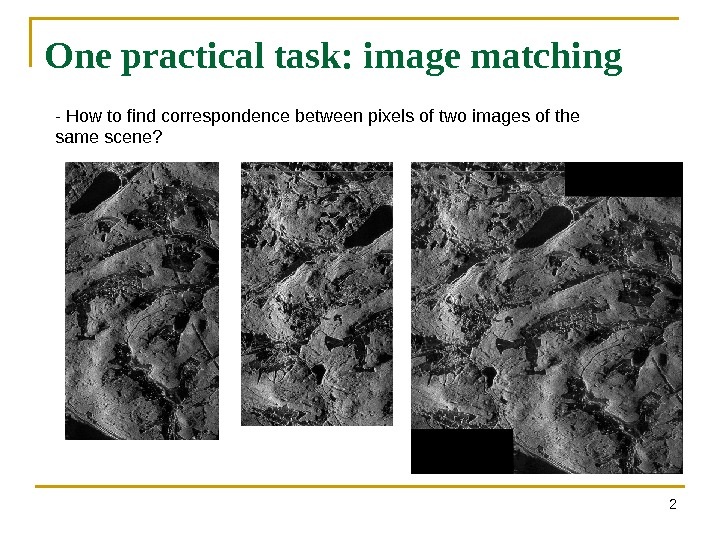One practical task: image matching 2 — How to find correspondence between pixels of two images of the same scene?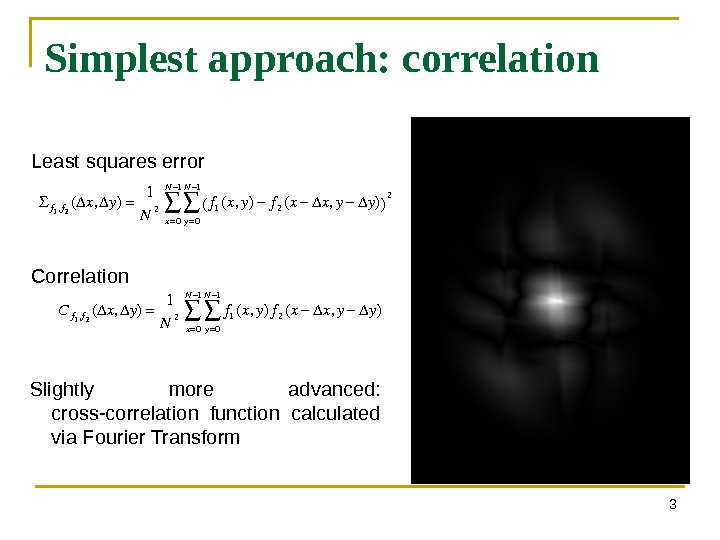3 Simplest approach: correlation Slightly more advanced: cross-correlation function calculated via Fourier Transform f 1 , f 2 ( Δ x , Δ y ) = 1 N 2 f 1 ( x , y ) − f 2 ( x − Δ x , y − Δ y ) ( ) 2 y = 0 N − 1 ∑ x = 0 N − 1 ∑Least squares error C f 1 , f 2 ( Δ x , Δ y ) = 1 N 2 f 1 ( x , y ) f 2 ( x − Δ x , y − Δ y ) y = 0 N − 1 ∑ x = 0 N − 1 ∑Correlation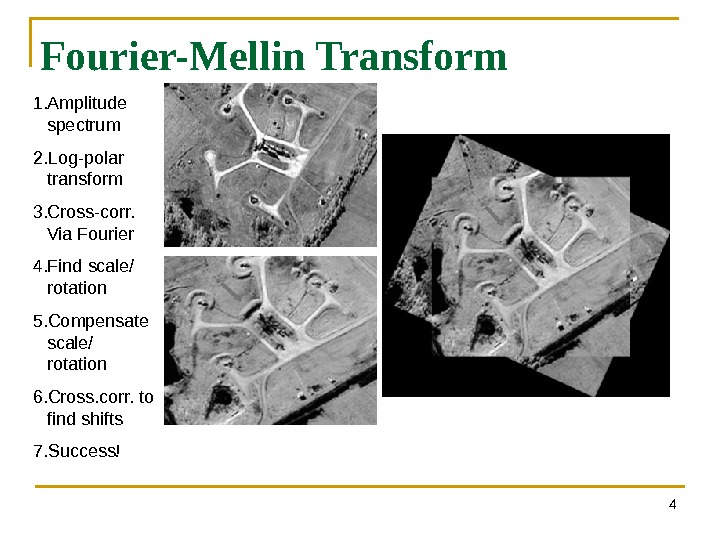Fourier-Mellin Transform 41. Amplitude spectrum 2. Log-polar transform 3. Cross-corr. Via Fourier 4. Find scale/ rotation 5. Compensate scale/ rotation 6. Cross. corr. to find shifts 7. Success!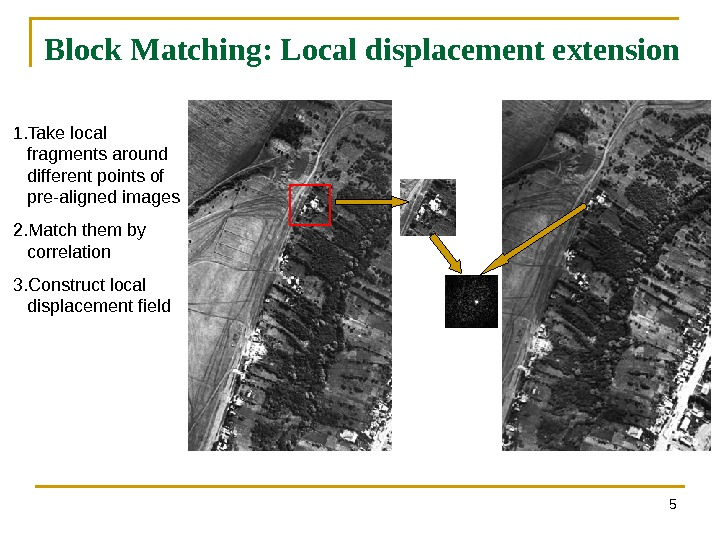Block Matching: Local displacement extension 51. Take local fragments around different points of pre-aligned images 2. Match them by correlation 3. Construct local displacement field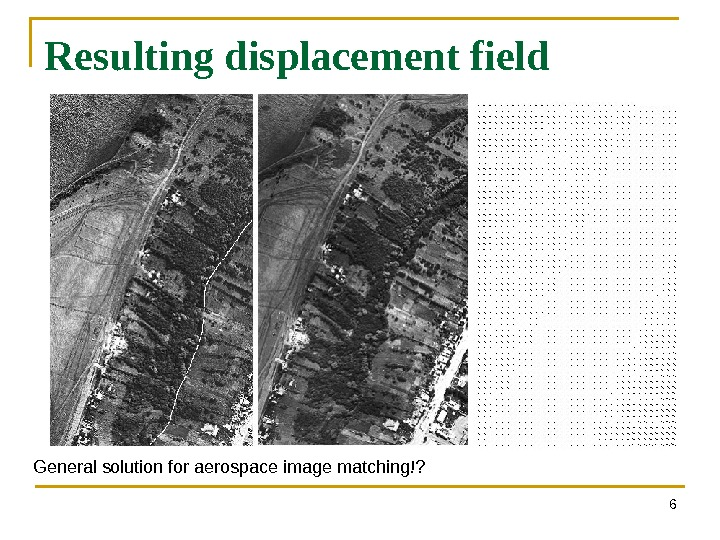Resulting displacement field 6 General solution for aerospace image matching!?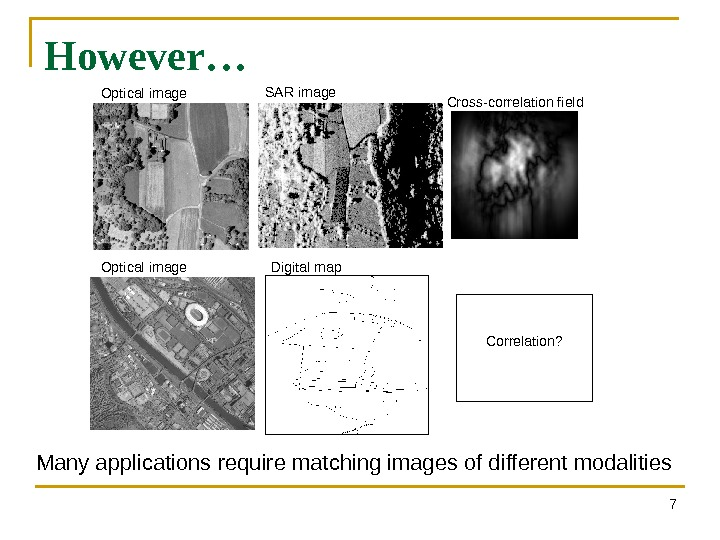However… 7 Optical image SAR image Cross-correlation field Many applications require matching images of different modalities Optical image Digital map Correlation?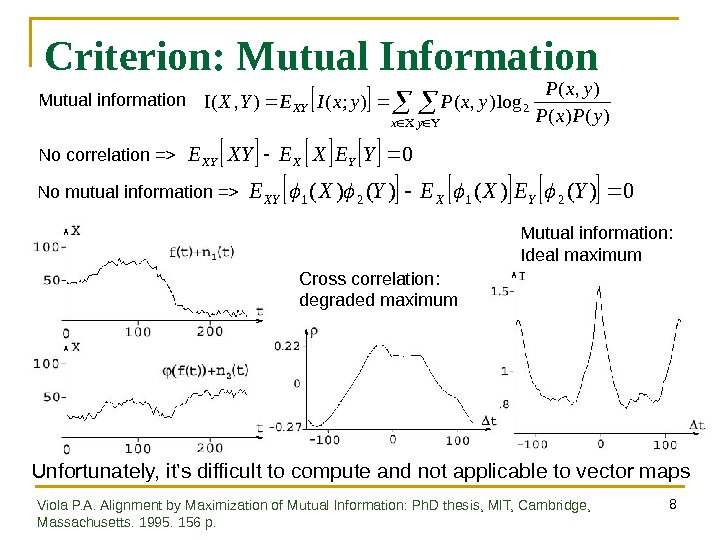8 Criterion: Mutual Information 0 YEXEXYEYXXY 0)()(2121 YEXEYXEYXXY No correlation => No mutual information => xy XY y. Px. P yx. Pyx. IEYX )()( ), ( log), (); (), (2 Mutual information Cross correlation: degraded maximum Mutual information: Ideal maximum Unfortunately, it’s difficult to compute and not applicable to vector maps Viola P. A. Alignment by Maximization of Mutual Information: Ph. D thesis, MIT, Cambridge, Massachusetts. 1995. 156 p.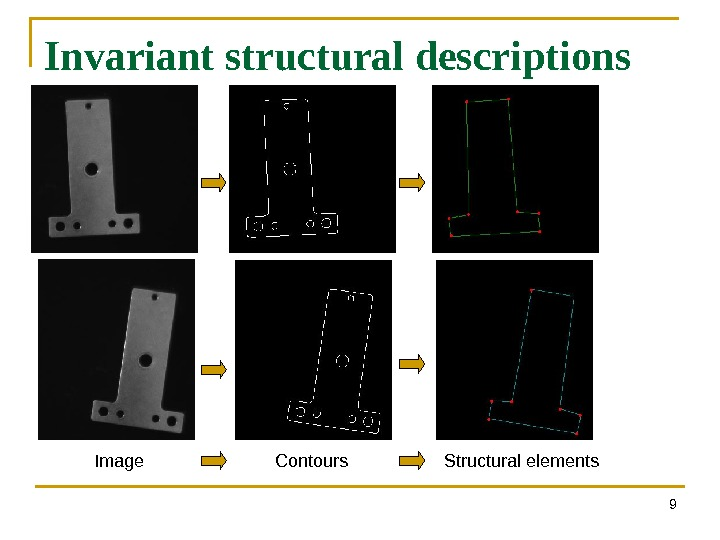Invariant structural descriptions 9 Image Contours Structural elements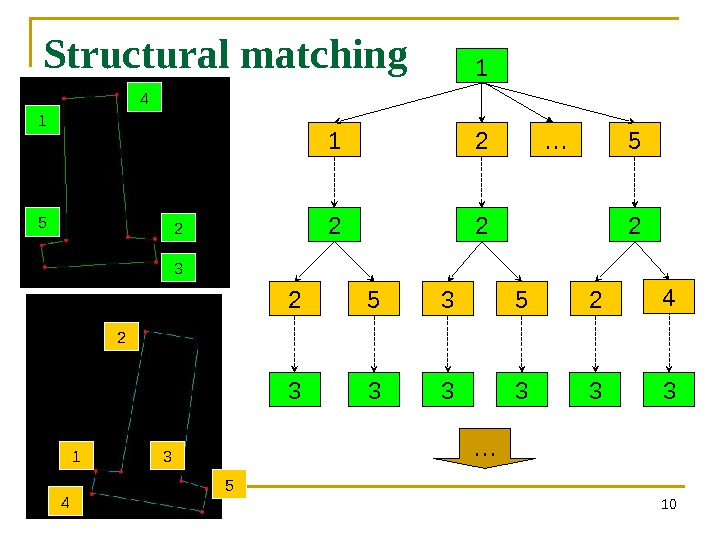Structural matching 101 2 5 1 2 3 52 2 424 2 31 5 1 2 3 4 5 … 3 3 3 …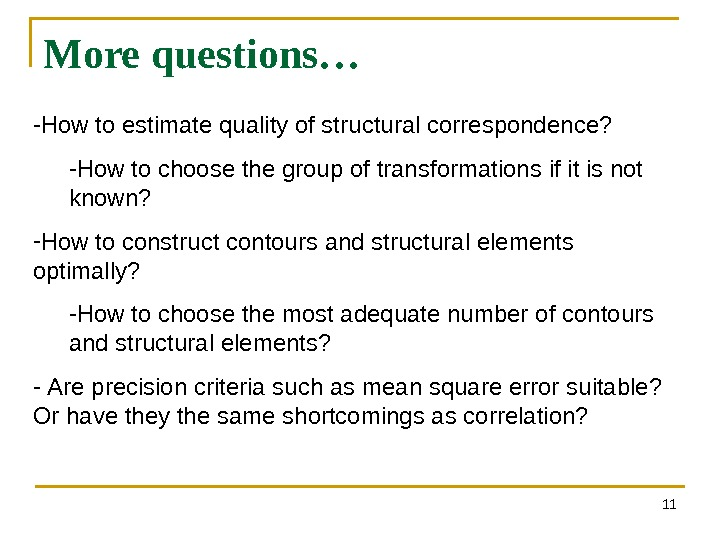More questions… 11 — How to estimate quality of structural correspondence? — How to choose the group of transformations if it is not known? — How to construct contours and structural elements optimally? — How to choose the most adequate number of contours and structural elements? — Are precision criteria such as mean square error suitable? Or have they the same shortcomings as correlation?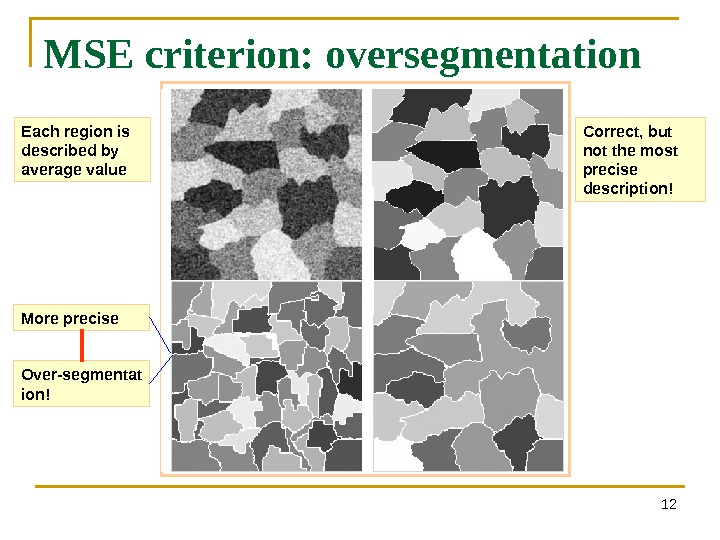MSE criterion: oversegmentation 12 More precise Over-segmentat ion! Each region is described by average value Correct, but not the most precise description!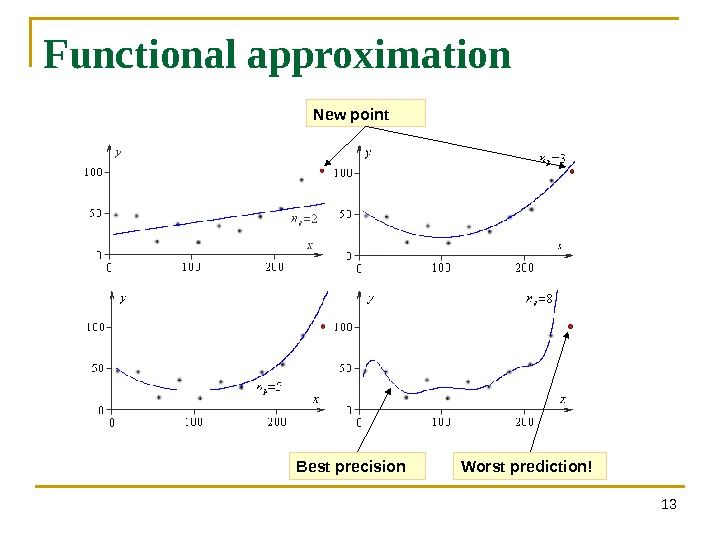Functional approximation 13 New point Worst prediction!Best precision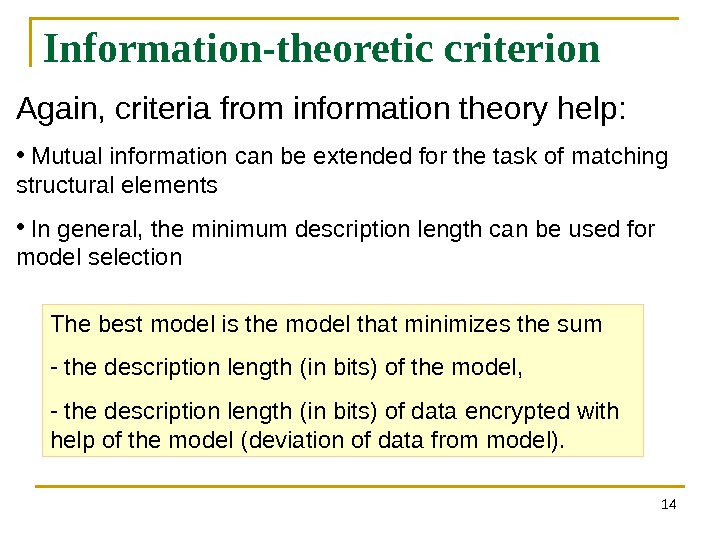Information-theoretic criterion 14 Again, criteria from information theory help: • Mutual information can be extended for the task of matching structural elements • In general, the minimum description length can be used for model selection The best model is the model that minimizes the sum — the description length ( in bits ) of the model , — the description length ( in bits ) of data encrypted with help of the model ( deviation of data from model ).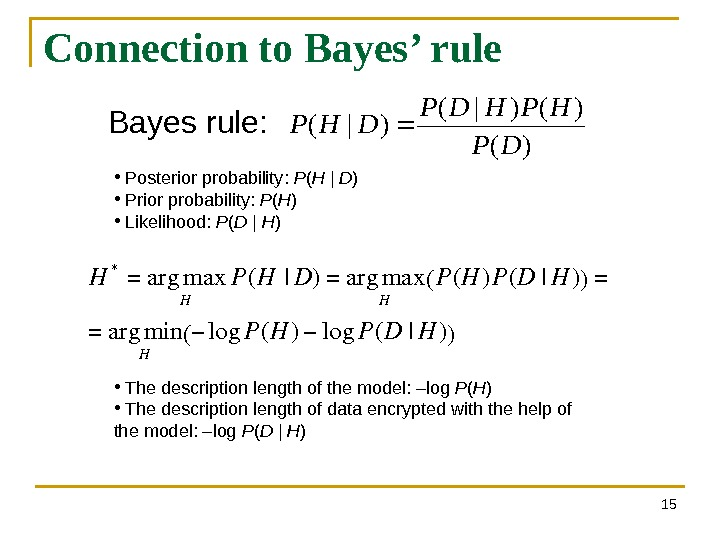Connection to Bayes’ rule 15)( )()|( DP HPHDP DHPBayes rule: • P osterior probability : P ( H | D ) • Prior probability: P ( H ) • Likelihood: P ( D | H ) H *=argmax HP(H|D)=argmax HP(H)P(D|H)()= =argmin H−log. P(H)−log. P(D|H)() • The description length of the model: –log P ( H ) • The description length of data encrypted with the help of the model: –log P ( D | H )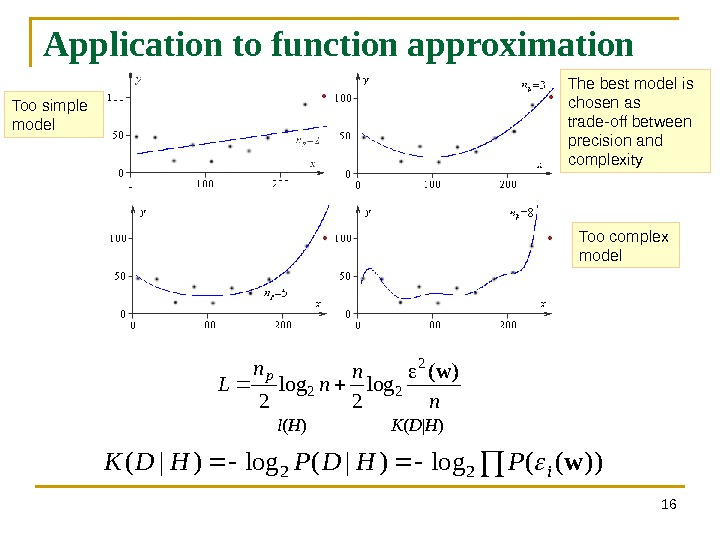Application to function approximation 16 n n Lp )( log 2 2 22 w l ( H ) K ( D | H ) ))((log)|( 22 w i. PHDPHDK Too simple model Too complex model. The best model is chosen as trade-off between precision and complexity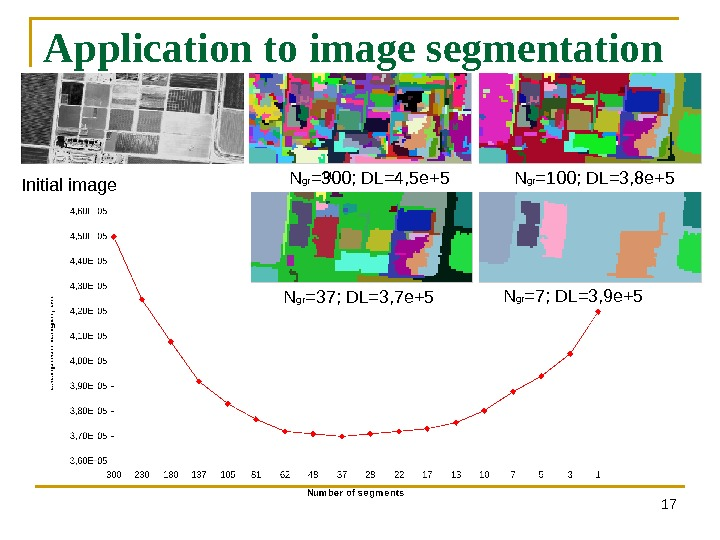Application to image segmentation 17 N gr =300; DL=4, 5 e+5 N gr =100; DL=3, 8 e+5 N gr =37; DL=3, 7 e+5 N gr =7; DL=3, 9 e+5 Initial image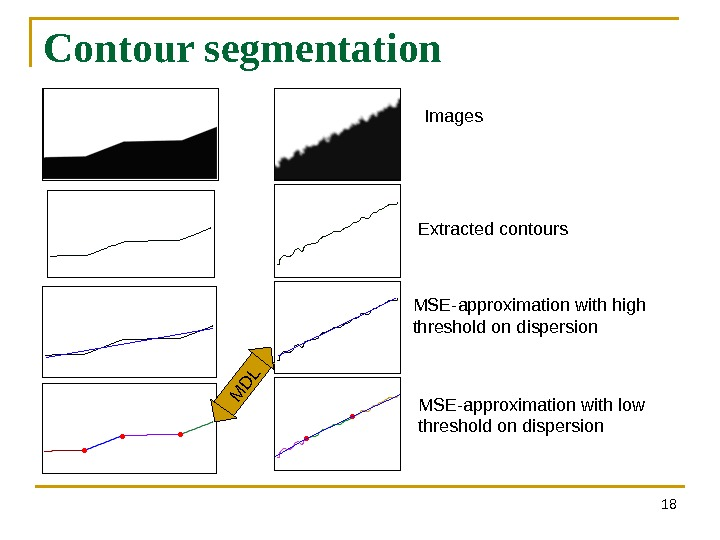Contour segmentation 18 M D LImages Extracted contours MSE-approximation with high threshold on dispersion MSE-approximation with low threshold on dispersion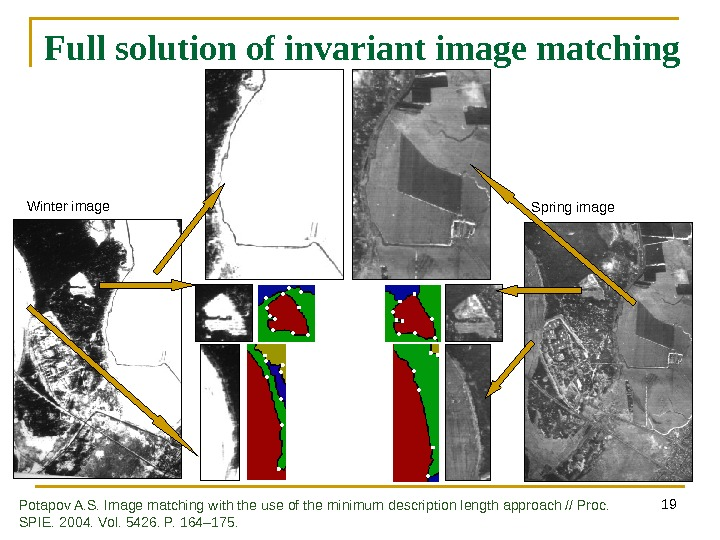19 Full solution of invariant image matching Winter image Spring image Potapov A. S. Image matching with the use of the minimum description length approach // Proc. SPIE. 2004. Vol. 5426. P. 164 – 175.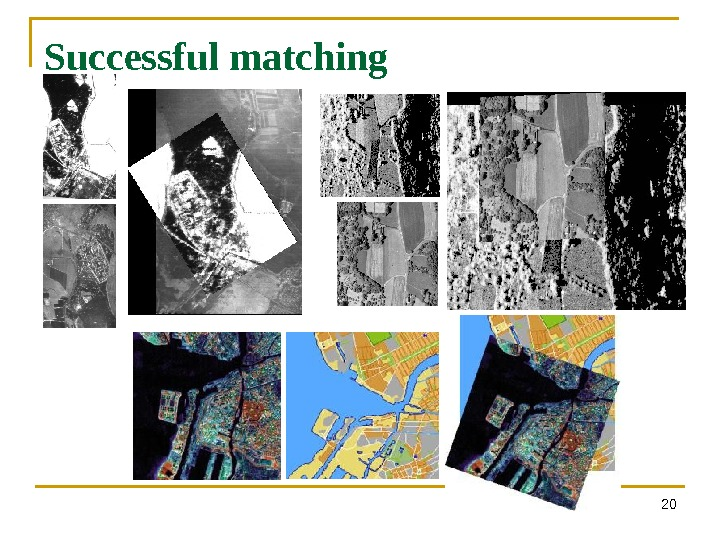20 Successful matching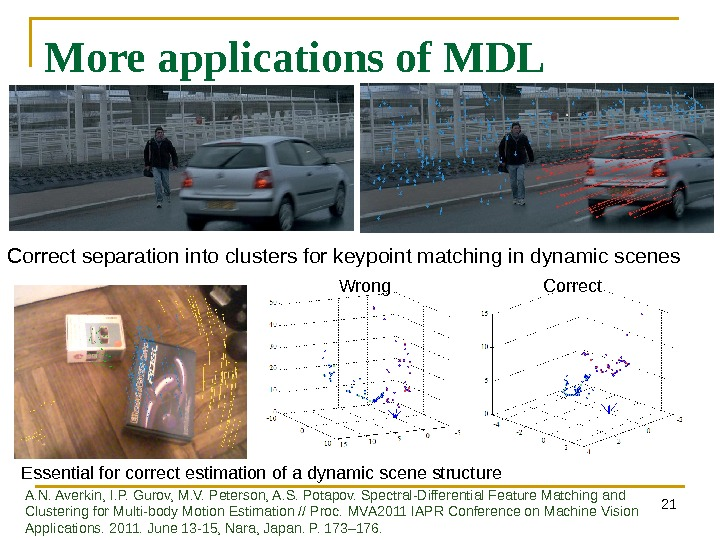21 More applications of MDL Correct separation into clusters for keypoint matching in dynamic scenes Essential for correct estimation of a dynamic scene structure Wrong Correct A. N. Averkin, I. P. Gurov, M. V. Peterson, A. S. Potapov. Spectral-Differential Feature Matching and Clustering for Multi-body Motion Estimation // Proc. MVA 2011 IAPR Conference on Machine Vision Applications. 2011. June 13 -15, Nara, Japan. P. 173– 176.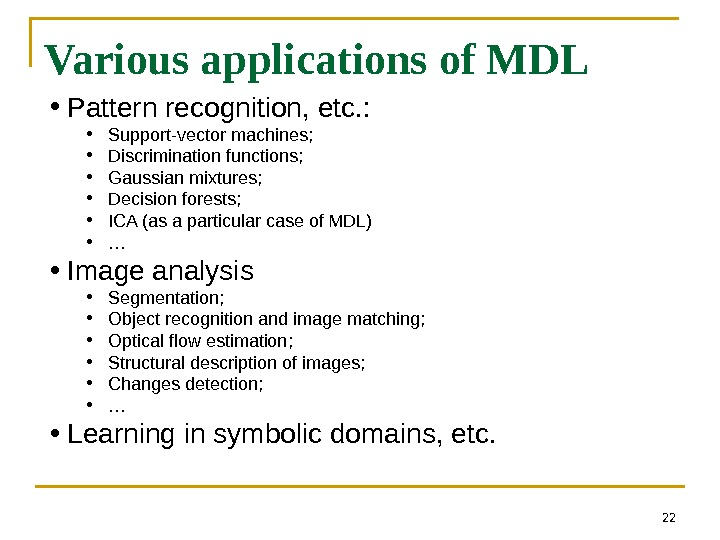22 • Pattern recognition, etc. : • Support-vector machines; • Discrimination functions; • Gaussian mixtures; • Decision forests; • ICA (as a particular case of MDL) • … • Image analysis • Segmentation; • Object recognition and image matching; • Optical flow estimation; • Structural description of images; • Changes detection; • … • Learning in symbolic domains, etc. Various applications of MDL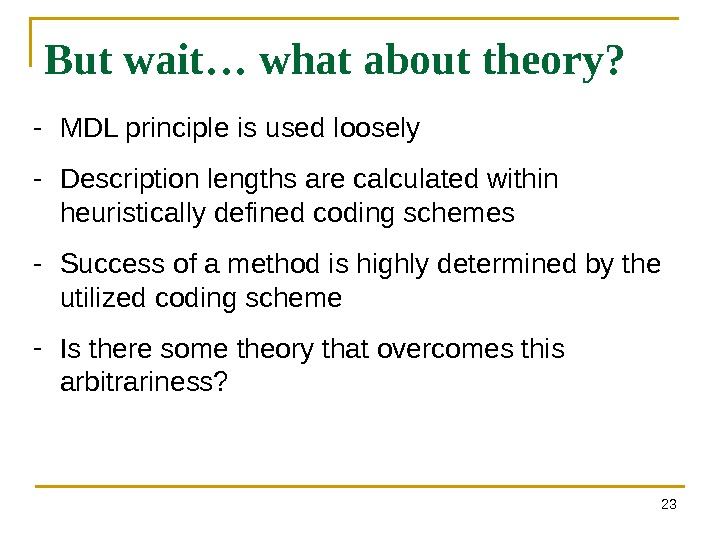23 But wait… what about theory? — MDL principle is used loosely — Description lengths are calculated within heuristically defined coding schemes — Success of a method is highly determined by the utilized coding scheme — Is there some theory that overcomes this arbitrariness?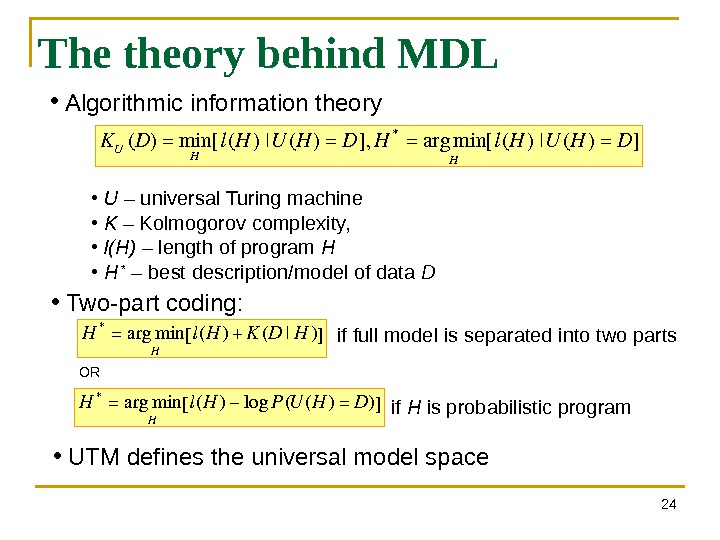24 The theory behind MDL • Algorithmic information theory KU(D)=min H [l(H)|U(H)=D], H*=argmin H [l(H)|U(H)=D] • U – universal Turing machine • K – Kolmogorov complexity, • l(H) – length of program H • H * – best description/model of data D H *=argmin Hl(H)+K(D|H)[] • Two-part coding: • UTM defines the universal model space H*=argmin H l(H)−log. P(U(H)=D)[] OR if H is probabilistic programif full model is separated into two parts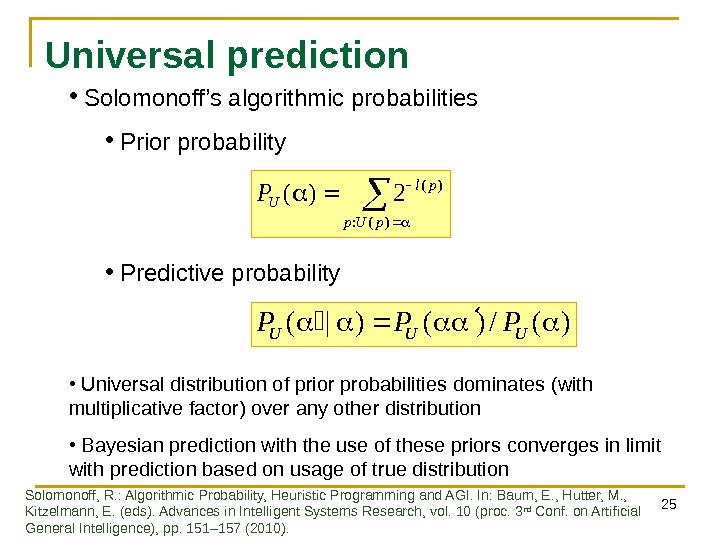25 Universal prediction )(: )( 2)( p. Up pl UP )(/)()|(UUUPPP • Solomonoff’s algorithmic probabilities • Prior probability • Predictive probability • Universal distribution of prior probabilities dominates ( with multiplicative factor ) over any other distribution • Bayesian prediction with the use of these priors converges in limit with prediction based on usage of true distribution Solomonoff, R. : Algorithmic Probability, Heuristic Programming and AGI. In: Baum, E. , Hutter, M. , Kitzelmann, E. (eds). Advances in Intelligent Systems Research, vol. 10 (proc. 3 rd Conf. on Artificial General Intelligence), pp. 151– 157 (2010).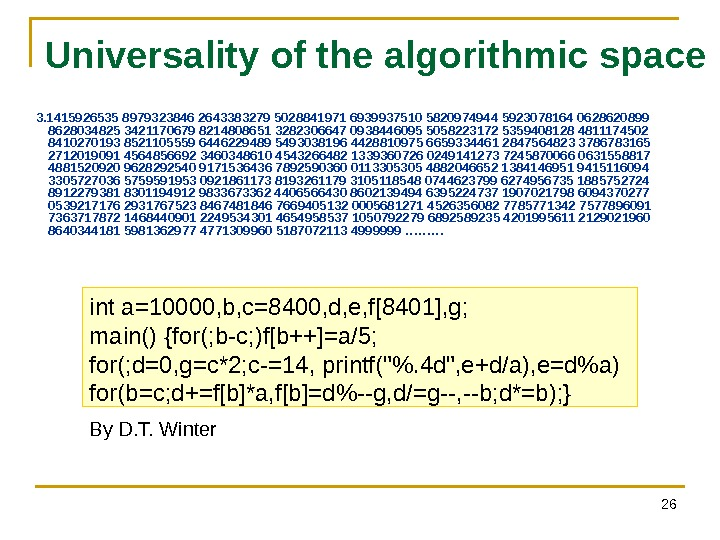26 Universality of the algorithmic space 3. 1415926535 8979323846 2643383279 5028841971 6939937510 5820974944 5923078164 0628620899 8628034825 3421170679 8214808651 3282306647 0938446095 5058223172 5359408128 4811174502 8410270193 8521105559 6446229489 5493038196 4428810975 6659334461 2847564823 3786783165 2712019091 4564856692 3460348610 4543266482 1339360726 0249141273 7245870066 0631558817 4881520920 9628292540 9171536436 7892590360 0113305305 4882046652 1384146951 9415116094 3305727036 5759591953 0921861173 8193261179 3105118548 0744623799 6274956735 1885752724 8912279381 8301194912 9833673362 4406566430 8602139494 6395224737 1907021798 6094370277 0539217176 2931767523 8467481846 7669405132 0005681271 4526356082 7785771342 7577896091 7363717872 1468440901 2249534301 4654958537 1050792279 6892589235 4201995611 2129021960 8640344181 5981362977 4771309960 5187072113 4 999999 ……… int a=10000, b, c=8400, d, e, f, g; main() {for(; b-c; )f[b++]=a/5; for(; d=0, g=c*2; c-=14, printf(«%. 4 d», e+d/a), e=d%a) for(b=c; d+=f[b]*a, f[b]=d%—g, d/=g—, —b; d*=b); } By D. T. Winter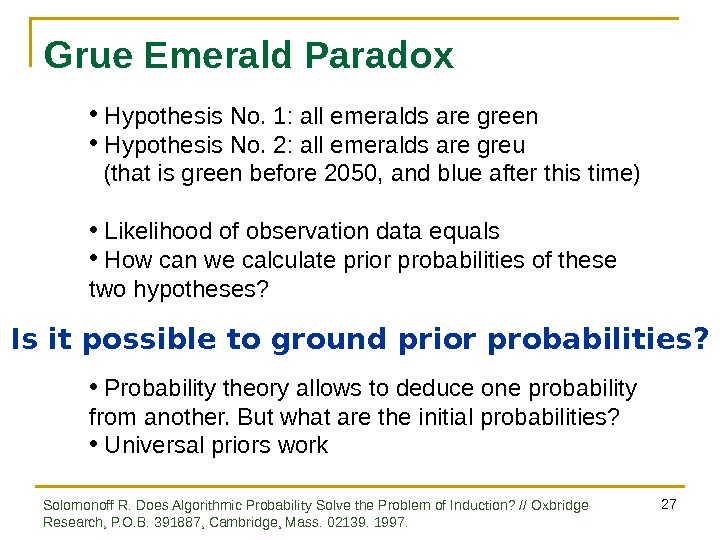27 Grue Emerald Paradox • Hypothesis No. 1: all emeralds are green • Hypothesis No. 2: all emeralds are greu (that is green before 2050, and blue after this time) • Likelihood of observation data equals • How can we calculate prior probabilities of these two hypotheses? Is it possible to ground prior probabilities? • Probability theory allows to deduce one probability from another. But what are the initial probabilities? • Universal priors work Solomonoff R. Does Algorithmic Probability Solve the Problem of Induction? // Oxbridge Research, P. O. B. 391887, Cambridge, Mass. 02139. 1997.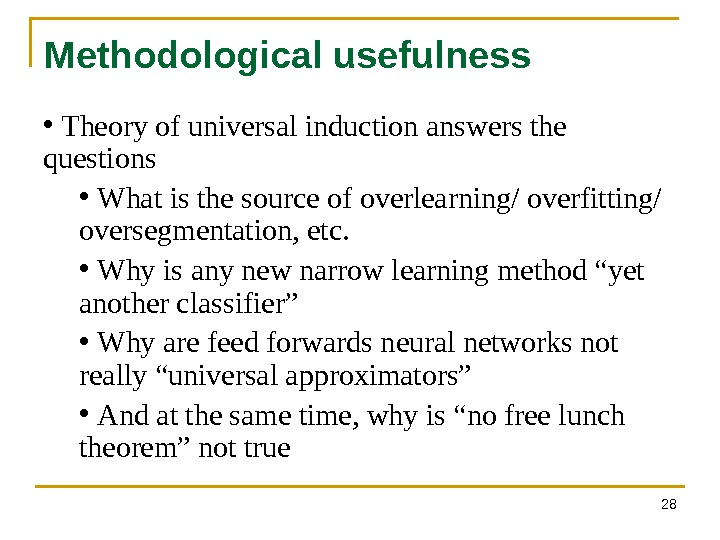28 Methodological usefulness • Theory of universal induction answers the questions • What is the source of overlearning/ overfitting/ oversegmentation, etc. • Why is any new narrow learning method “yet another classifier” • Why are feed forwards neural networks not really “universal approximators” • And at the same time, why is “no free lunch theorem” not true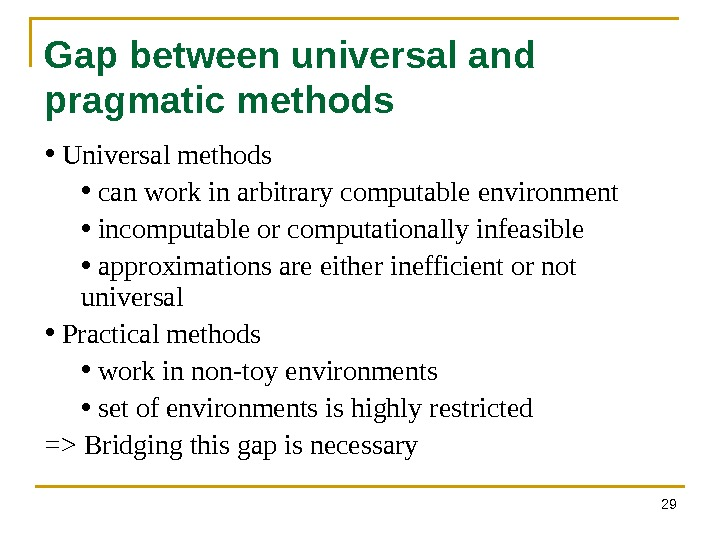29 Gap between universal and pragmatic methods • Universal methods • can work in arbitrary computable environment • incomputable or computationally infeasible • approximations are either inefficient or not universal • Practical methods • work in non-toy environments • set of environments is highly restricted => Bridging this gap is necessary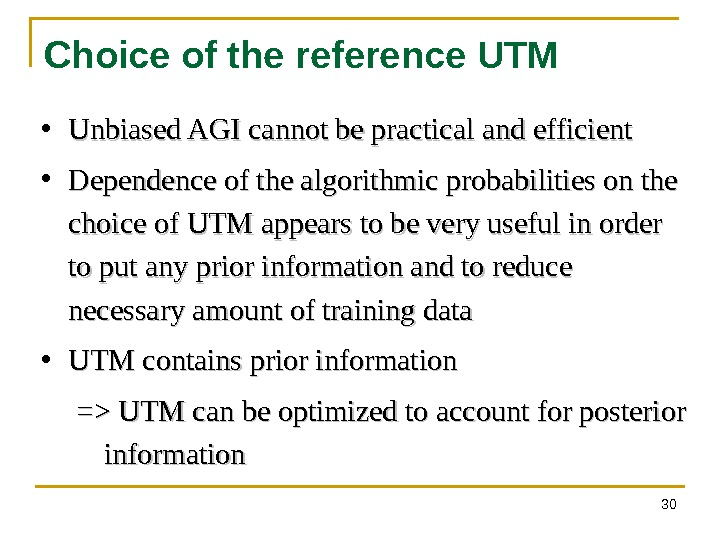30 Choice of the reference UTM • Unbiased AGI cannot be practical and efficient • Dependence of the algorithmic probabilities on the choice of UTM appears to be very useful in order to put any prior information and to reduce necessary amount of training data • UTM contains prior information => UTM can be optimized to account for posterior information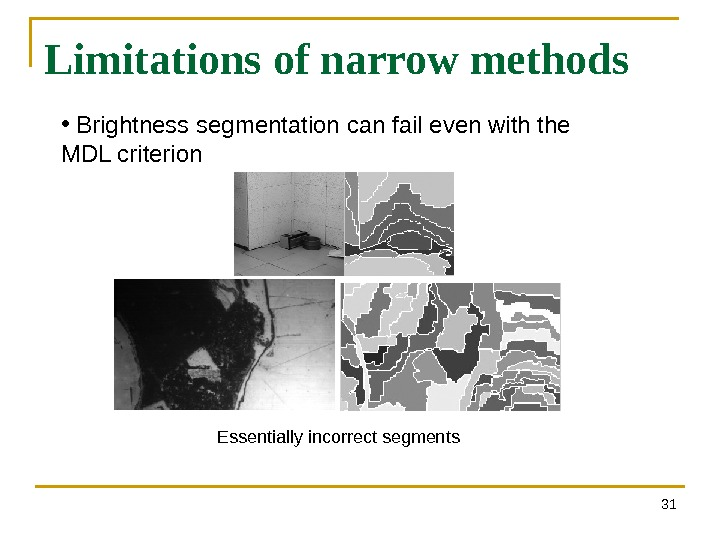31 Limitations of narrow methods • Brightness segmentation can fail even with the MDL criterion Essentially incorrect segments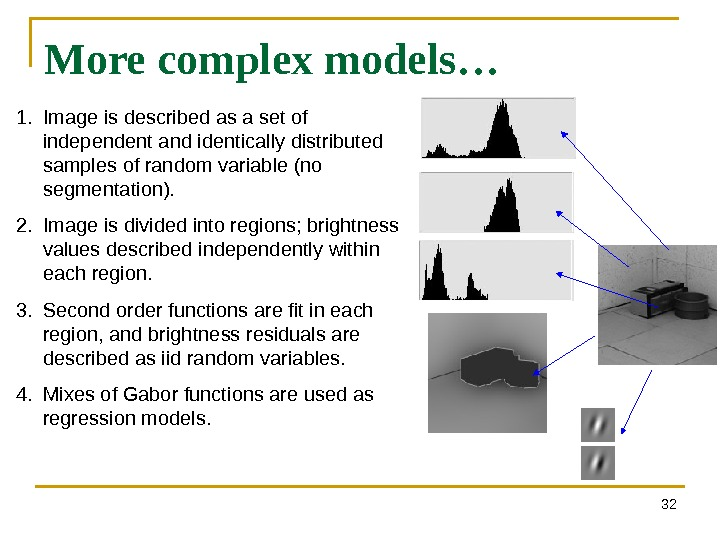32 More complex models… 1. Image is described as a set of independent and identically distributed samples of random variable (no segmentation). 2. Image is divided into regions; brightness values described independently within each region. 3. Second order functions are fit in each region, and brightness residuals are described as iid random variables. 4. Mixes of Gabor functions are used as regression models.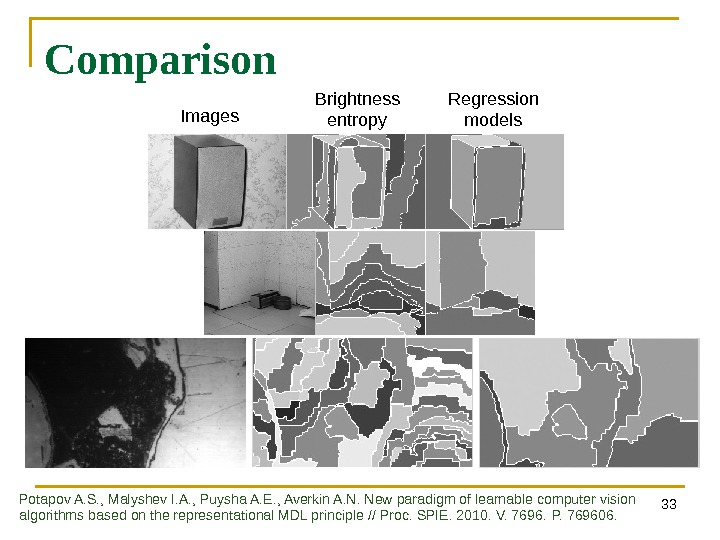33 Comparison Images Brightness entropy Regression models Potapov A. S. , Malyshev I. A. , Puysha A. E. , Averkin A. N. New paradigm of learnable computer vision algorithms based on the representational MDL principle // Proc. SPIE. 2010. V. 7696. P. 769606.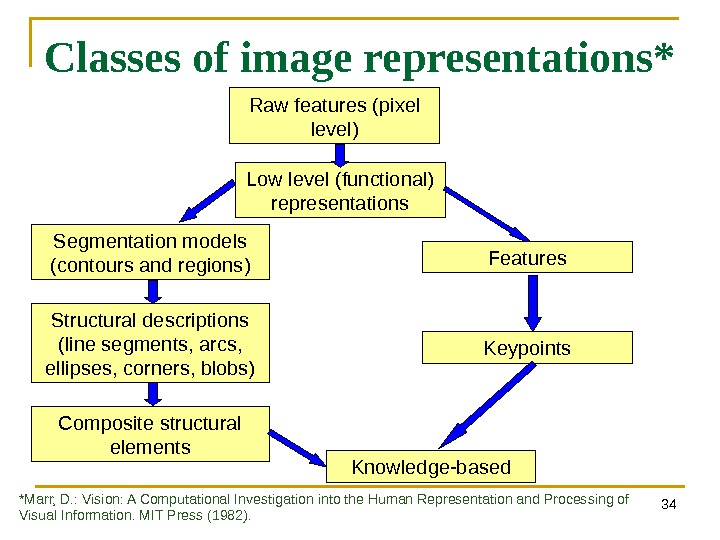34 Classes of image representations* Low level (functional) representations. Raw features (pixel level) Segmentation models ( contours and regions ) Structural descriptions ( line segments , arcs , ellipses , corners , blobs ) Features Keypoints Composite structural elements Knowledge-based *Marr, D. : Vision: A Computational Investigation into the Human Representation and Processing of Visual Information. MIT Press (1982).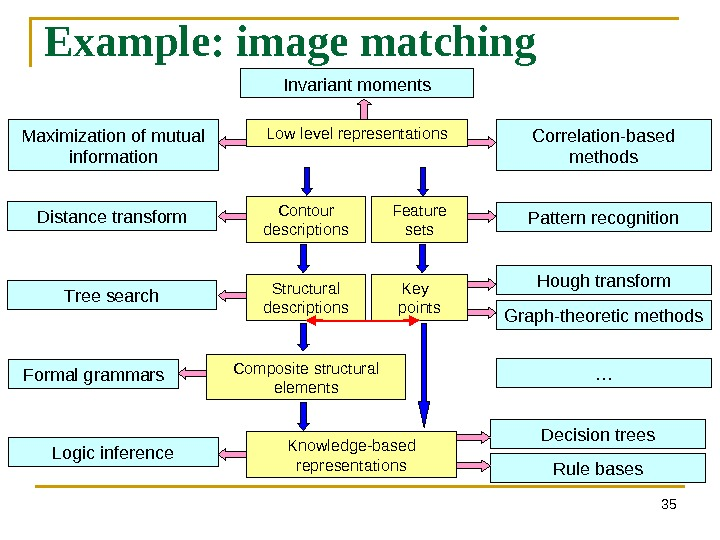35 Example: image matching Low level representations Contour descriptions Structural descriptions Feature sets Key points Composite structural elements Knowledge-based representations Correlation-based methods. Maximization of mutual information Invariant moments Distance transform Pattern recognition Tree search Formal grammars Hough transform Graph-theoretic methods Decision trees Rule bases. Logic inference …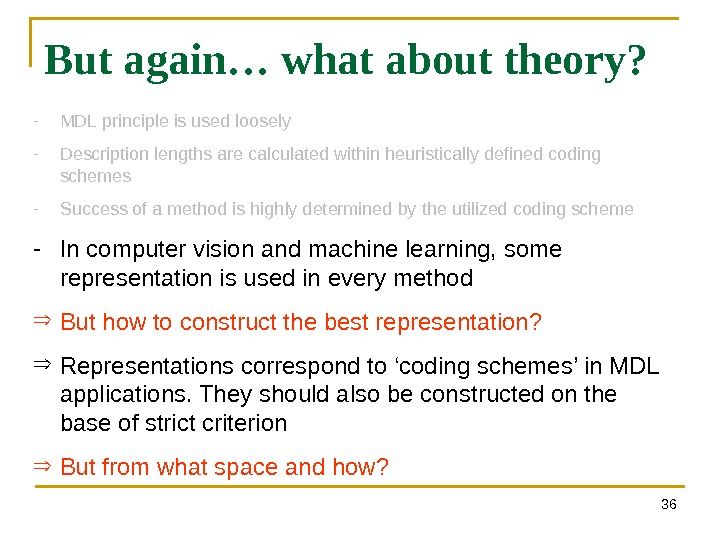36 But again… what about theory? — MDL principle is used loosely — Description lengths are calculated within heuristically defined coding schemes — Success of a method is highly determined by the utilized coding scheme — In computer vision and machine learning, some representation is used in every method But how to construct the best representation? Representations correspond to ‘coding schemes’ in MDL applications. They should also be constructed on the base of strict criterion But from what space and how?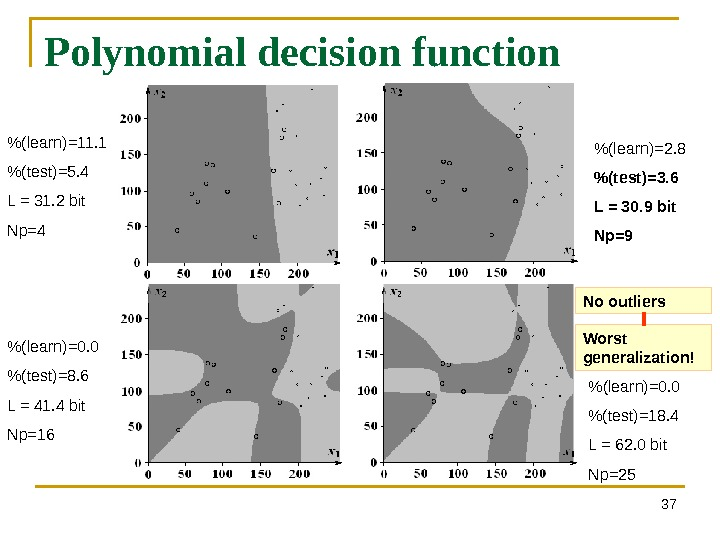37 Polynomial decision function % (learn)=11. 1 %(test)=5. 4 L = 31. 2 bit Np=4 % (learn)=2. 8 %(test)=3. 6 L = 30. 9 bit Np=9 % (learn)=0. 0 %(test)=8. 6 L = 41. 4 bit Np=16 % (learn)=0. 0 %(test)=18. 4 L = 62. 0 bit Np=25 No outliers Worst generalization!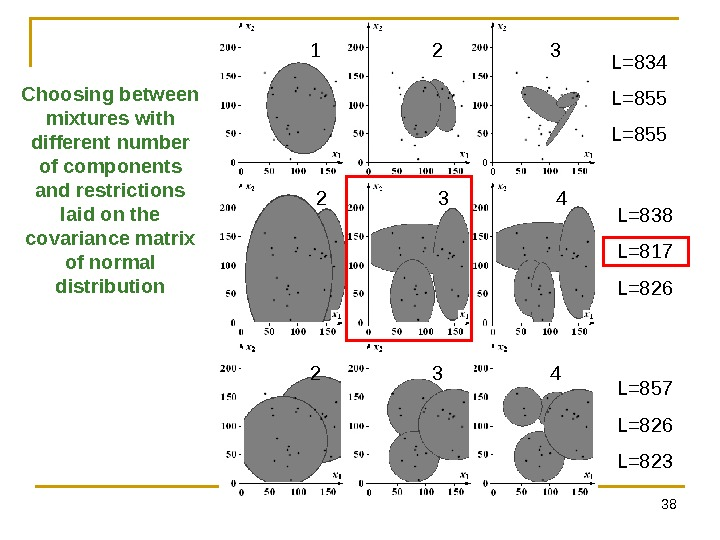38 Choosing between mixtures with different number of components and restrictions laid on the covariance matrix of normal distribution L=834 L=855 L=838 L=817 L=826 L=857 L=826 L=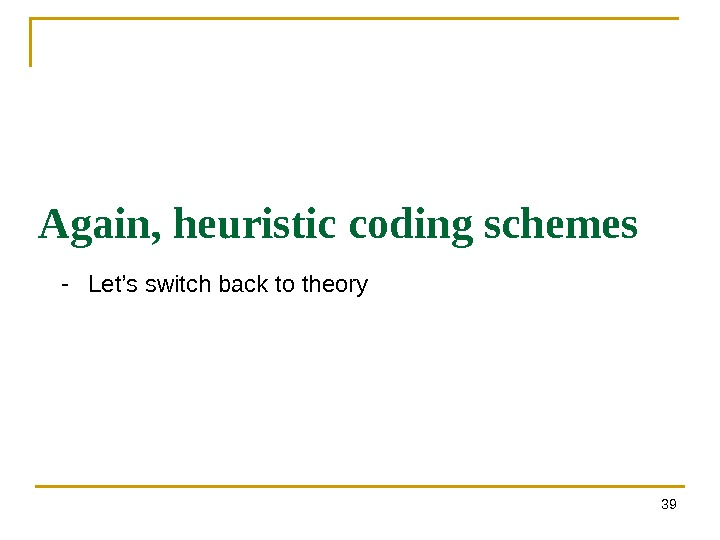39 Again, heuristic coding schemes — Let’s switch back to theory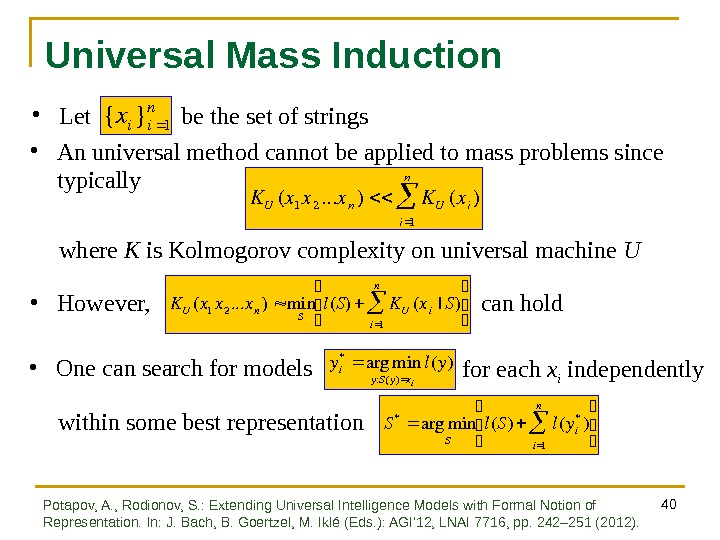40 Universal Mass Induction • Let be the set of strings {xi}i 1 n KU(x 1 x 2. . . xn)KU(xi) i 1 n • An universal method cannot be applied to mass problems since typically where K is Kolmogorov complexity on universal machine U • However, can hold K U(x 1 x 2. . . x n)min Sl(S)K U(x i|S) i 1 n • One can search for models y i* arg min y : S ( y ) x i l ( y ) S * arg min S l ( S ) l ( y i* ) i 1 n within some best representation for each x i independently Potapov, A. , Rodionov, S. : Extending Universal Intelligence Models with Formal Notion of Representation. In: J. Bach, B. Goertzel, M. Iklé (Eds. ): AGI’ 12, LNAI 7716, pp. 242– 251 (2012).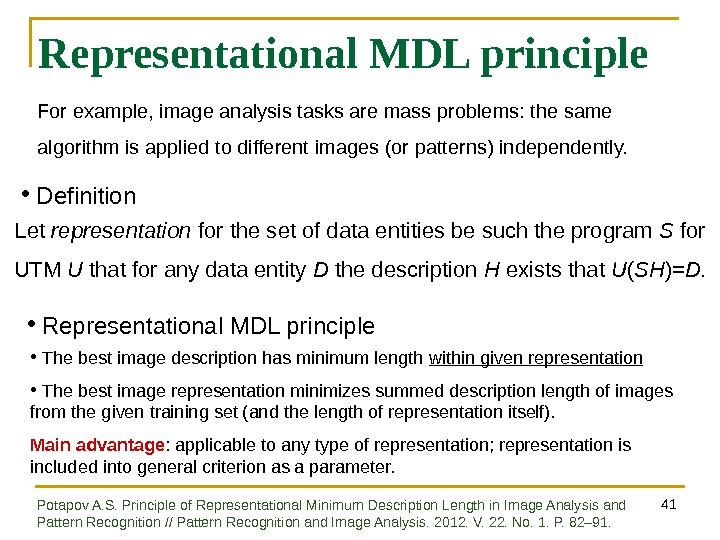41 Representational MDL principle • Definition Let representation for the set of data entities be such the program S for UTM U that for any data entity D the description H exists that U ( SH )= D. • Representational MDL principle • The best image description has minimum length within given representation • The best image representation minimizes summed description length of images from the given training set (and the length of representation itself). Main advantage : applicable to any type of representation; representation is included into general criterion as a parameter. For example, image analysis tasks are mass problems: the same algorithm is applied to different images (or patterns) independently. Potapov A. S. Principle of Representational Minimum Description Length in Image Analysis and Pattern Recognition // Pattern Recognition and Image Analysis. 2012. V. 22. No. 1. P. 82– 91.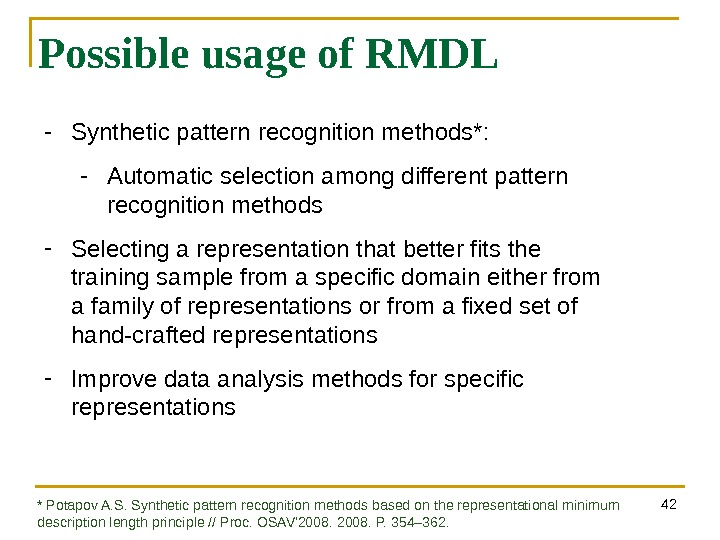42 Possible usage of RMDL — Synthetic pattern recognition methods*: — Automatic selection among different pattern recognition methods — Selecting a representation that better fits the training sample from a specific domain either from a family of representations or from a fixed set of hand-crafted representations — Improve data analysis methods for specific representations * Potapov A. S. Synthetic pattern recognition methods based on the representational minimum description length principle // Proc. OSAV’2008. P. 354– 362.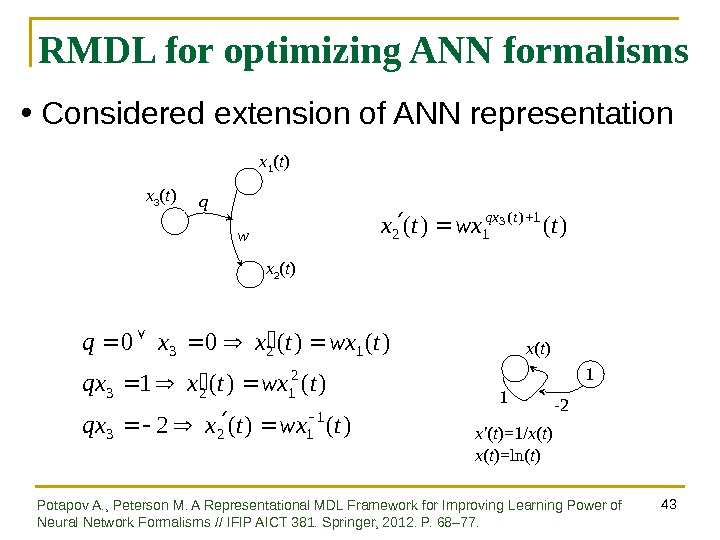43 RMDL for optimizing ANN formalisms x 3 ( t ) x 1 ( t ) w x 2 ( t )q)()( 12 3 twxtx tqx )()(2 )()(1 )()(00 1 123 2 123 twxtxqx twxtxxq x ( t ) 1 x’ ( t )=1/ x ( t )=ln( t ) -2 1 • Considered extension of ANN representation Potapov A. , Peterson M. A Representational MDL Framework for Improving Learning Power of Neural Network Formalisms // IFIP AICT 381. Springer, 2012. P. 68– 77.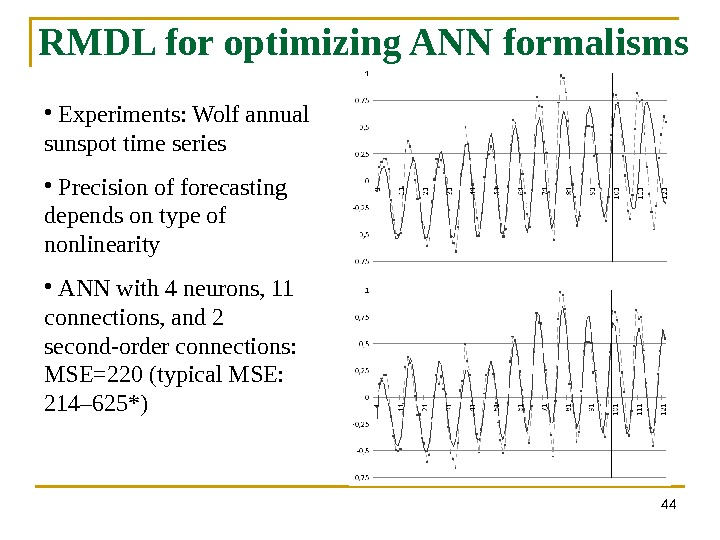44 RMDL for optimizing ANN formalisms • Experiments: Wolf annual sunspot time series • Precision of forecasting depends on type of nonlinearity • ANN with 4 neurons, 11 connections, and 2 second-order connections: MSE=220 (typical MSE: 214– 625*)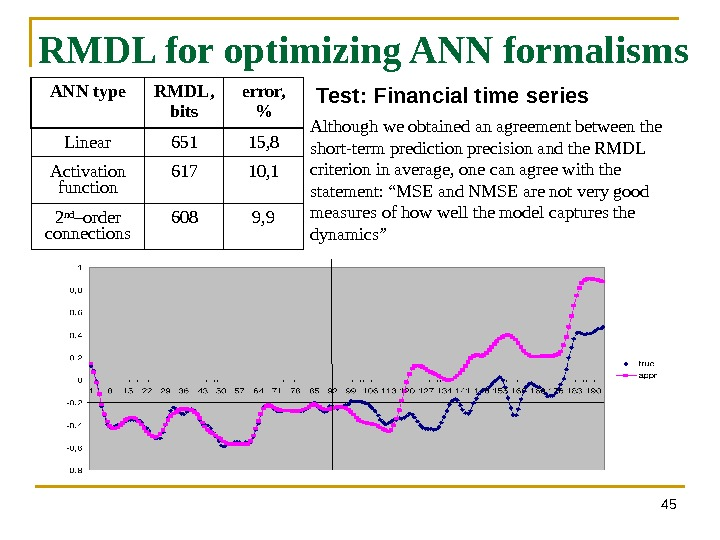45 RMDL for optimizing ANN formalisms ANN type RMDL, bits error, % Linear 651 15, 8 Activation function 617 10, 1 2 nd –order connections 608 9, 9 Although we obtained an agreement between the short-term prediction precision and the RMDL criterion in average, one can agree with the statement: “MSE and NMSE are not very good measures of how well the model captures the dynamics” Test: Financial time series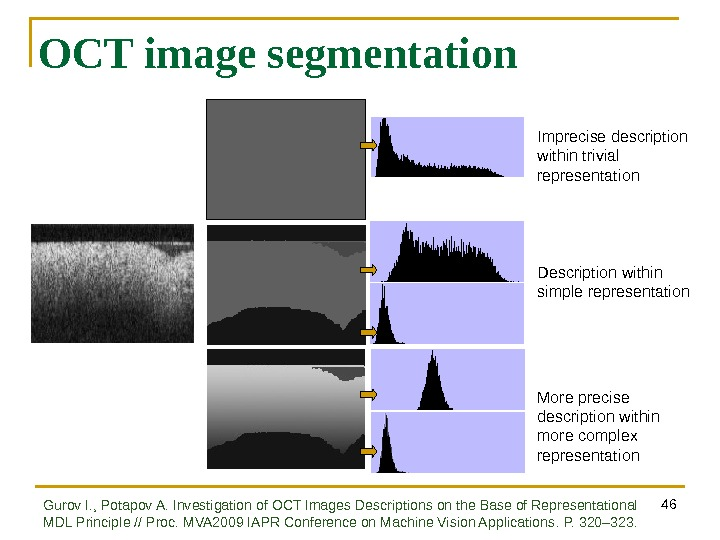46 OCT image segmentation Imprecise description within trivial representation Description within simple representation More precise description within more complex representation Gurov I. , Potapov A. Investigation of OCT Images Descriptions on the Base of Representational MDL Principle // Proc. MVA 2009 IAPR Conference on Machine Vision Applications. P. 320 – 323.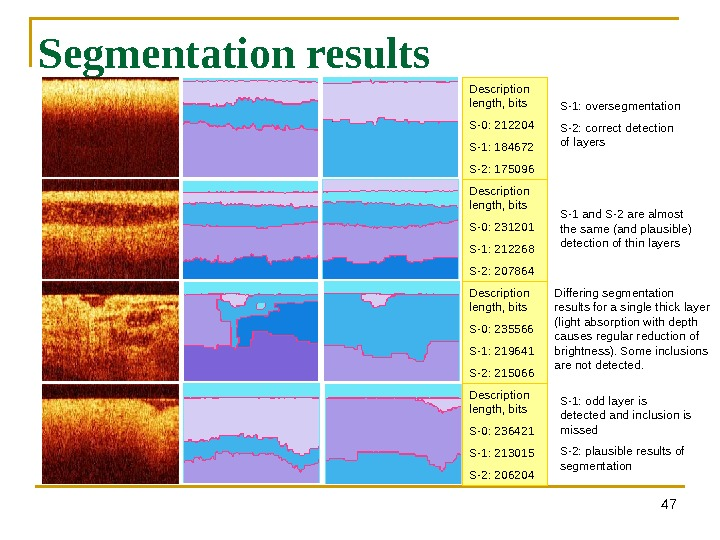47 Segmentation results Description length, bits S-0: 212204 S-1: 184672 S-2: 175096 Description length, bits S-0: 231201 S-1: 212268 S-2: 207864 Description length, bits S-0: 235566 S-1: 219641 S-2: 215066 Description length, bits S-0: 236421 S-1: 213015 S-2: 206204 S-1: oversegmentation S-2: correct detection of layers S-1 and S-2 are almost the same (and plausible) detection of thin layers Differing segmentation results for a single thick layer (light absorption with depth causes regular reduction of brightness). Some inclusions are not detected. S-1: odd layer is detected and inclusion is missed S-2: plausible results of segmentation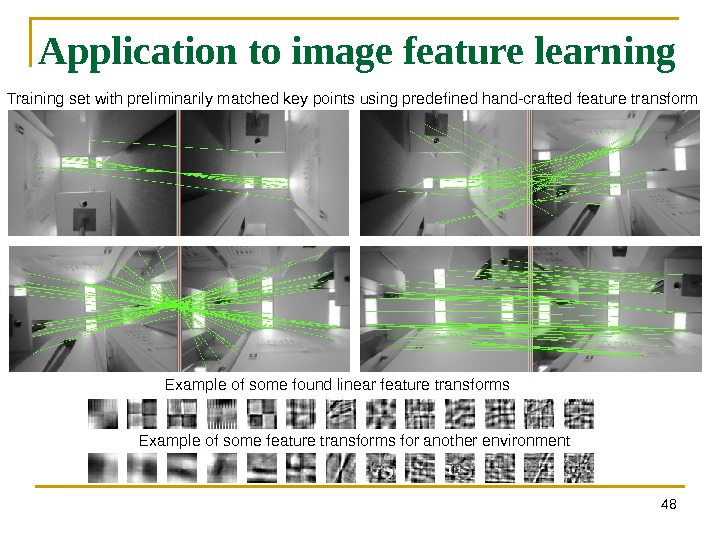48 Application to image feature learning Training set with preliminarily matched key points using predefined hand-crafted feature transform Example of some found linear feature transforms Example of some feature transforms for another environment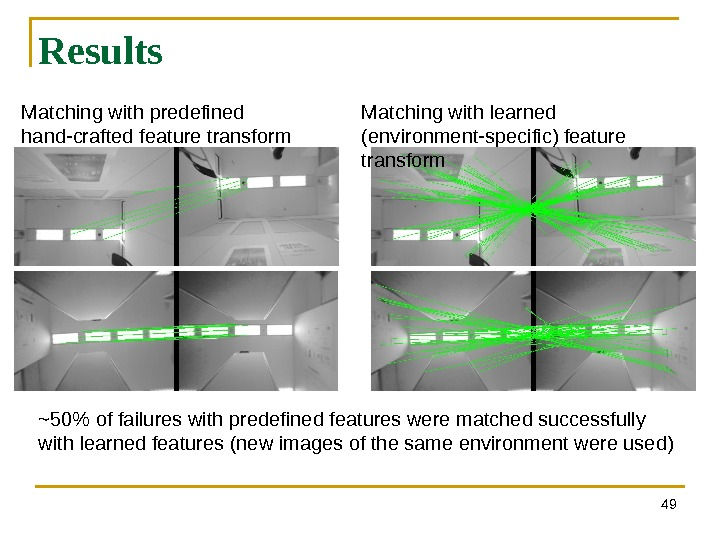49 Results Matching with predefined hand-crafted feature transform Matching with learned (environment-specific) feature transform ~50% of failures with predefined features were matched successfully with learned features (new images of the same environment were used)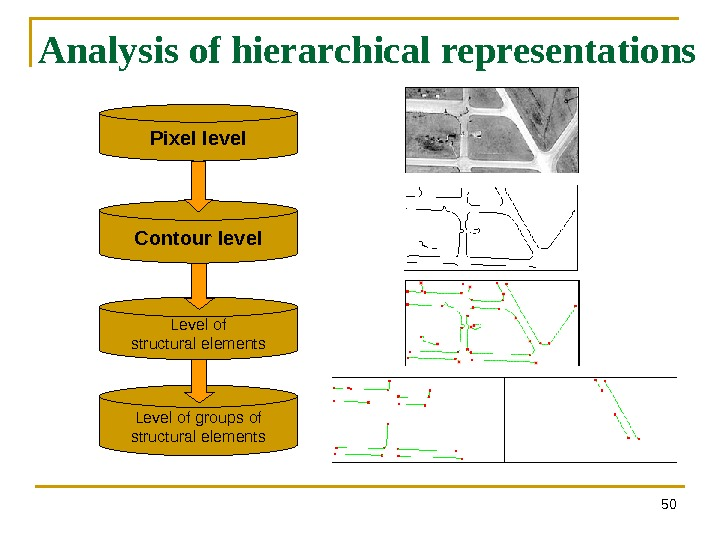50 Analysis of hierarchical representations Pixel level Contour level Level of structural elements Level of groups of structural elements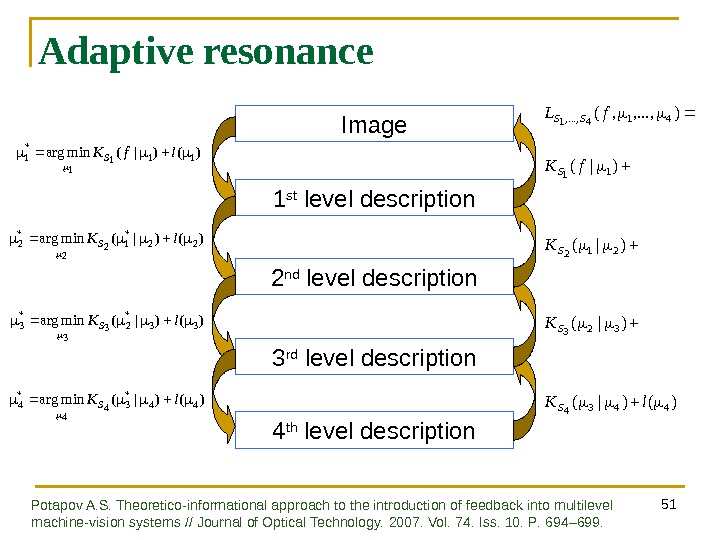51 Adaptive resonance Image 1 st level description 2 nd level description 3 rd level description)()|(minarg 33* 2 3 3* 3 l. K S )()|(minarg 22* 1 2 2* 2 l. K S )()|(minarg 44* 3 4 4* 4 l. K S )()|( )|( ), . . . , , ( 443 4 32 3 21 2 1 1 41 4, . . . , 1 l. KKK f. L SSSS SS )()|(minarg 11 1 1* 1 lf. K S 4 th level description Potapov A. S. Theoretico-informational approach to the introduction of feedback into multilevel machine-vision systems // Journal of Optical Technology. 2007. Vol. 74. Iss. 10. P. 694 – 699.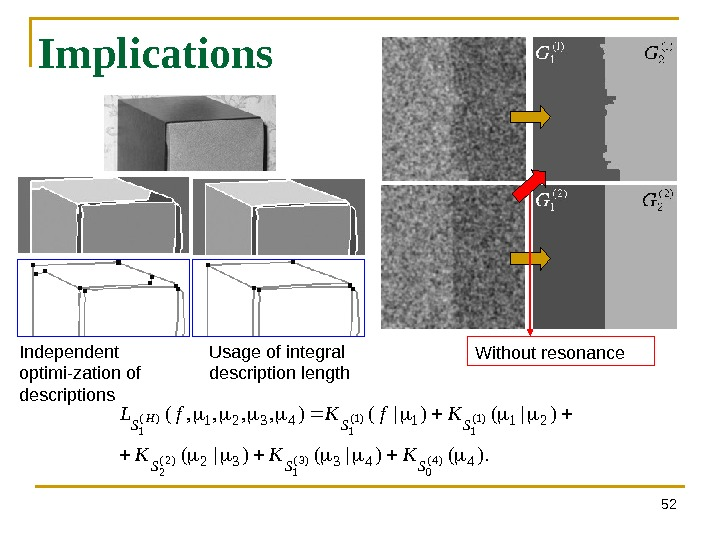52 Implications ). ()|()|(), , ( 44332 2114321 )4( 0)3( 1)2( 2 )1( 1)( 1 SSS KKK Kf. L HIndependent optimi-zation of descriptions Usage of integral description length Without resonance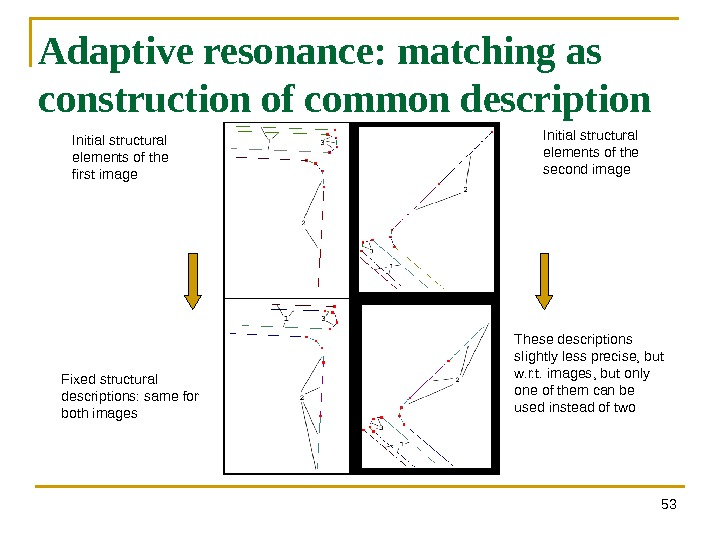53 Adaptive resonance: matching as construction of common description Initial structural elements of the first image Initial structural elements of the second image Fixed structural descriptions: same for both images These descriptions slightly less precise, but w. r. t. images, but only one of them can be used instead of two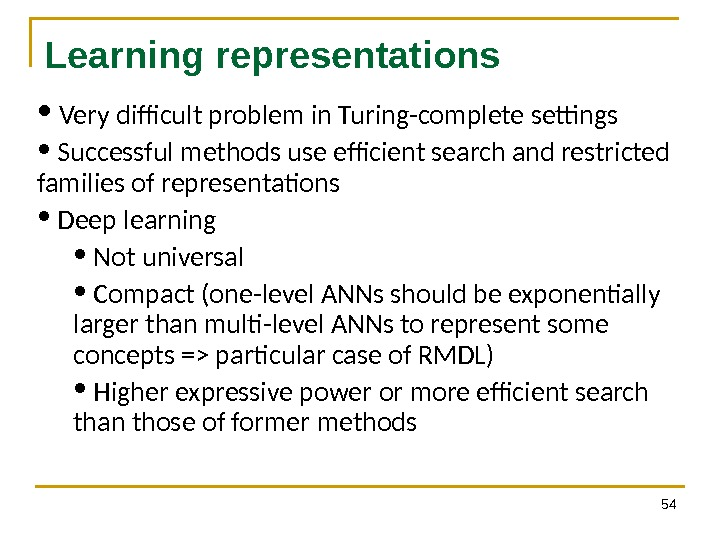54 Learning representations • Very difficult problem in Turing-complete settings • Successful methods use efficient search and restricted families of representations • Deep learning • Not universal • Compact (one-level ANNs should be exponentially larger than multi-level ANNs to represent some concepts => particular case of RMDL) • Higher expressive power or more efficient search than those of former methods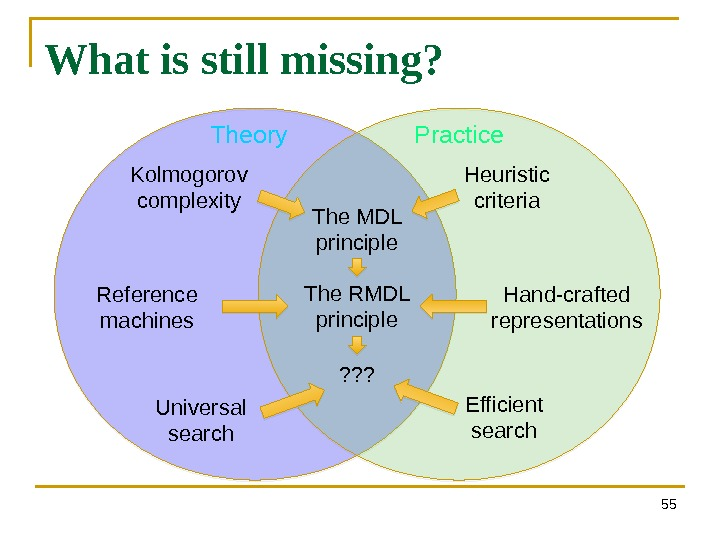55 What is still missing? The MDL principle The RMDL principle ? ? ? Kolmogorov complexity Heuristic criteria Reference machines Hand-crafted representations Efficient search. Universal search Theory Practice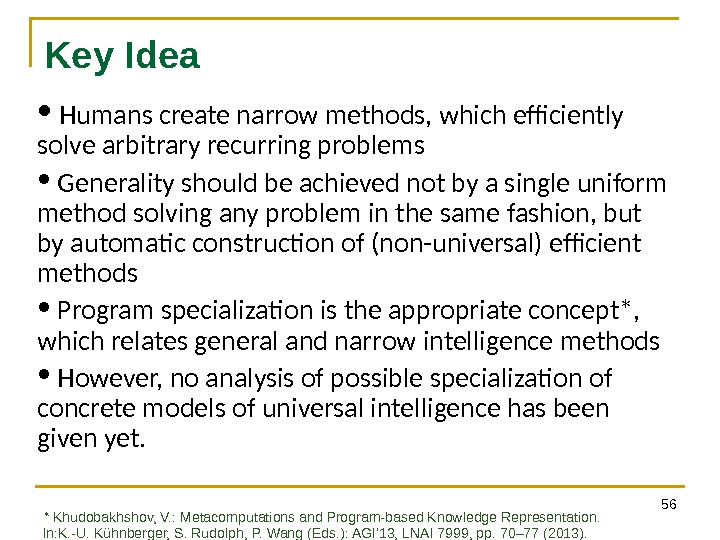56 Key Idea • Humans create narrow methods, which efficiently solve arbitrary recurring problems • Generality should be achieved not by a single uniform method solving any problem in the same fashion, but by automatic construction of (non-universal) efficient methods • Program specialization is the appropriate concept*, which relates general and narrow intelligence methods • However, no analysis of possible specialization of concrete models of universal intelligence has been given yet. * Khudobakhshov, V. : Metacomputations and Program-based Knowledge Representation. In: K. -U. Kühnberger, S. Rudolph, P. Wang (Eds. ): AGI’ 13, LNAI 7999, pp. 70– 77 (2013).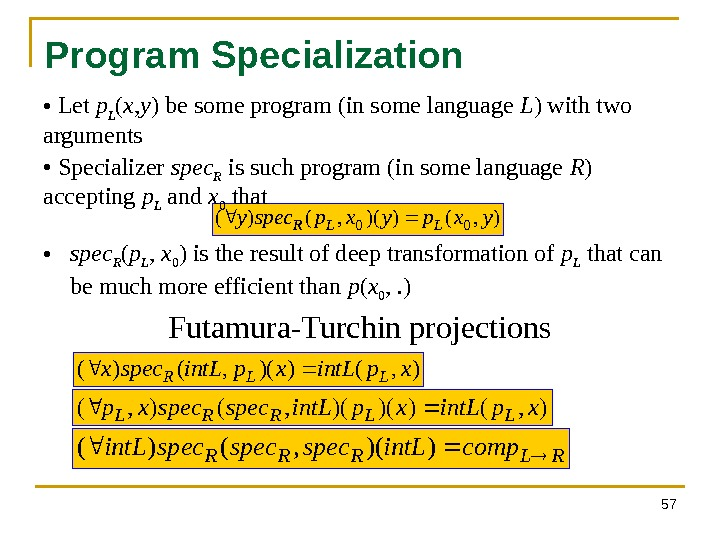57 Program Specialization), ())(, ()(00 yxpyxpspecy. LLR ), ())(, ()( xpint. Lspecx LLR ), ())()(, (), (xpint. Lspecxp. LLRRL RLRRRcompint. Lspecspecint. L))(, ()( • spec R ( p L , x 0 ) is the result of deep transformation of p L that can be much more efficient than p ( x 0 , . ) • Let p L ( x , y ) be some program (in some language L ) with two arguments • Specializer spec R is such program (in some language R ) accepting p L and x 0 that Futamura-Turchin projections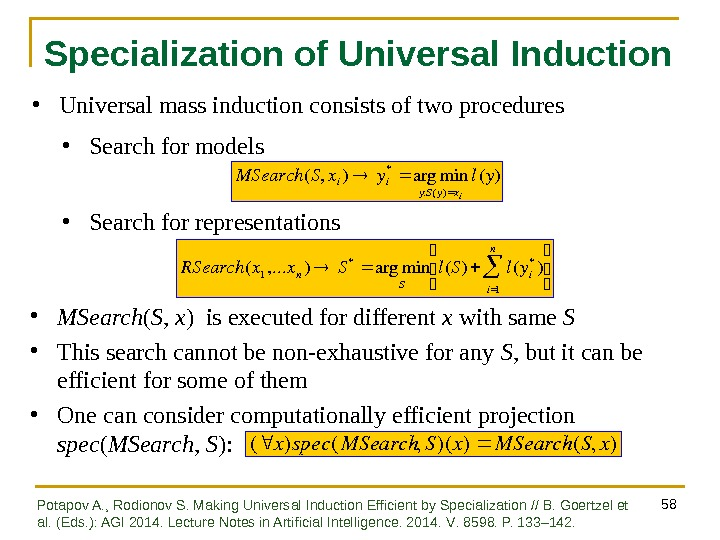58 Specialization of Universal Induction RSearch ( x 1 , . . . x n ) S * arg min S l ( S ) l ( y i* ) i 1 n MSearch(S, xi)yi *argmin y: S(y)xi l(y) • MSearch ( S , x ) is executed for different x with same S • This search cannot be non-exhaustive for any S , but it can be efficient for some of them • One can consider computationally efficient projection spec ( MSearch , S ): (x)spec(MSearch, S)(x)MSearch(S, x) • Universal mass induction consists of two procedures • Search for models • Search for representations Potapov A. , Rodionov S. Making Universal Induction Efficient by Specialization // B. Goertzel et al. (Eds. ): AGI 2014. Lecture Notes in Artificial Intelligence. 2014. V. 8598. P. 133– 142.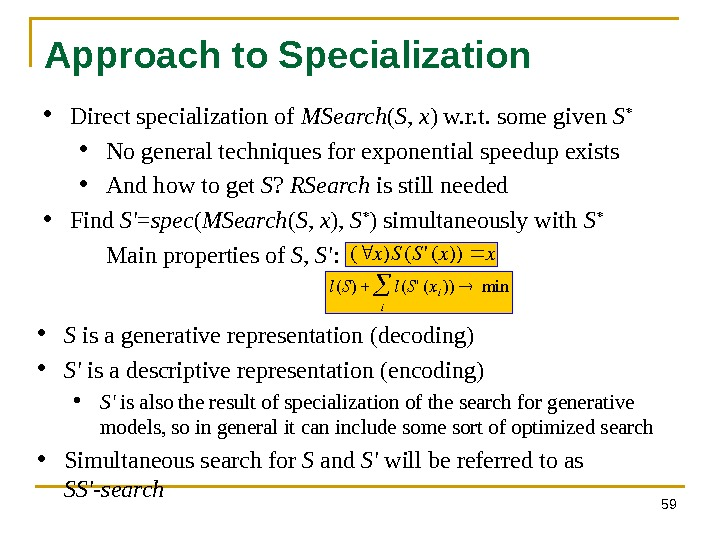59 Approach to Specialization • Direct specialization of MSearch ( S , x ) w. r. t. some given S * • No general techniques for exponential speedup exists • And how to get S ? RSearch is still needed • Find S’ = spec ( MSearch ( S , x ), S * ) simultaneously with S * • Main properties of S , S’ : (x)S(S'(x))x l ( S ) l ( S ‘ ( x i )) i min • S is a generative representation (decoding) • S’ is a descriptive representation (encoding) • S’ is also the result of specialization of the search for generative models, so in general it can include some sort of optimized search • Simultaneous search for S and S’ will be referred to as SS’-search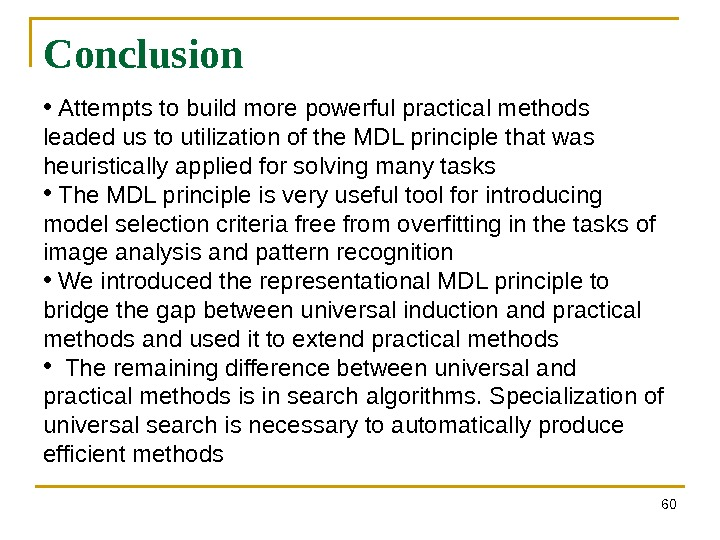Conclusion 60 • Attempts to build more powerful practical methods leaded us to utilization of the MDL principle that was heuristically applied for solving many tasks • The MDL principle is very useful tool for introducing model selection criteria free from overfitting in the tasks of image analysis and pattern recognition • We introduced the representational MDL principle to bridge the gap between universal induction and practical methods and used it to extend practical methods • The remaining difference between universal and practical methods is in search algorithms. Specialization of universal search is necessary to automatically produce efficient methods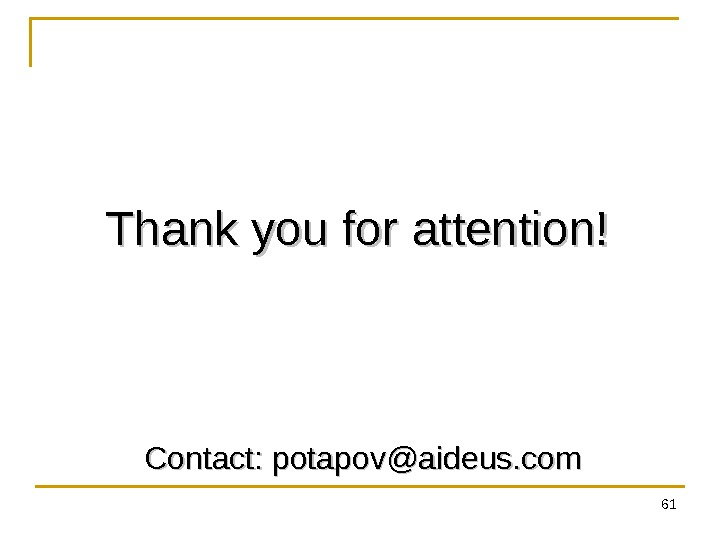61 Thank you for attention! Contact: [email protected] com